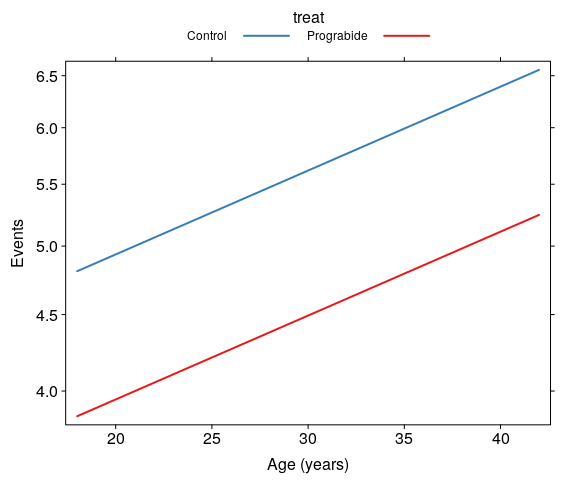# 1 Introduction

The aim of this vignette is to illustrate the use/functionality of the glm_coef function. glm_coef can be used to display model coefficients with confidence intervals and p-values. The advantages and limitations of glm_coef are:

1. Recognises the main models used in epidemiology/public health.
2. Automatically back transforms estimates and confidence intervals, when the model requires it.
3. Can use robust standard errors for the calculation of confidence intervals.
• Standard errors are used by default.
• The use of standard errors is restricted by the following classes of objects (models): gee, glm and survreg.
4. Can display nice labels for the names of the parameters.
5. Returns a data frame that can be modified and/or exported as tables for publications (with further editing).

We start by loading relevant packages and setting the theme for the plots (as suggested in the Template of this package):

rm(list = ls())
library(effects, warn.conflicts = FALSE)
library(latex2exp, warn.conflicts = FALSE)
library(multcomp, warn.conflicts = FALSE)
library(pander, warn.conflicts = FALSE)
library(papeR, warn.conflicts = FALSE)
library(pubh, warn.conflicts = FALSE)

panderOptions("table.split.table", Inf)
set.alignment("right", row.names = "left", permanent = TRUE)
trellis.par.set(tactile.theme())

# 2 Multiple Linear Regression

For continuous outcomes there is no need of exponentiating the results.

data(birthwt)
birthwt$smoke <- factor(birthwt$smoke, labels=c("Non-smoker", "Smoker"))
birthwt$race <- factor(birthwt$race > 1, labels=c("White", "Non-white"))
model_norm <- glm(bwt ~ smoke + race, data = birthwt)

Anova(model_norm)
#> Analysis of Deviance Table (Type II tests)
#>
#> Response: bwt
#>       LR Chisq Df Pr(>Chisq)
#> smoke   15.833  1  6.917e-05 ***
#> race    18.492  1  1.706e-05 ***
#> ---
#> Signif. codes:  0 '***' 0.001 '**' 0.01 '*' 0.05 '.' 0.1 ' ' 1
summary(model_norm)
#>
#> Call:
#> glm(formula = bwt ~ smoke + race, data = birthwt)
#>
#> Deviance Residuals:
#>      Min        1Q    Median        3Q       Max
#> -2313.82   -440.72     15.67    493.77   1655.18
#>
#> Coefficients:
#>               Estimate Std. Error t value Pr(>|t|)
#> (Intercept)    3334.82      91.16  36.582  < 2e-16 ***
#> smokeSmoker    -428.49     107.69  -3.979 9.90e-05 ***
#> raceNon-white  -452.10     105.13  -4.300 2.75e-05 ***
#> ---
#> Signif. codes:  0 '***' 0.001 '**' 0.01 '*' 0.05 '.' 0.1 ' ' 1
#>
#> (Dispersion parameter for gaussian family taken to be 471136.9)
#>
#>     Null deviance: 99969656  on 188  degrees of freedom
#> Residual deviance: 87631472  on 186  degrees of freedom
#> AIC: 3010.2
#>
#> Number of Fisher Scoring iterations: 2

Table of coefficients:

glm_coef(model_norm)
#>                              Coefficient Pr(>|t|)
#> (Intercept)   3334.82 (3013.71, 3655.93)  < 0.001
#> smokeSmoker   -428.49 (-677.74, -179.24)  < 0.001
#> raceNon-white  -452.1 (-759.05, -145.15)    0.004

Once we know the order in which the parameters are displayed, we can add labels to our final table:

Note: Compare results using naive and robust standard errors.

pander(glm_coef(model_norm, labels = c("Constant", "Smoker - Non-smoker",
"Non-white - White"), se.rob = FALSE),
caption = "Table of coeffients using naive standard errors.")
Table of coeffients using naive standard errors.
Coefficient Pr(>|t|)
Constant 3334.82 (3154.98, 3514.66) < 0.001
Smoker - Non-smoker -428.49 (-640.93, -216.05) < 0.001
Non-white - White -452.1 (-659.51, -244.69) < 0.001
pander(glm_coef(model_norm, labels = c("Constant", "Smoker - Non-smoker",
"Non-white - White")),
caption = "Table of coeffients using robust standard errors.")
Table of coeffients using robust standard errors.
Coefficient Pr(>|t|)
Constant 3334.82 (3013.71, 3655.93) < 0.001
Smoker - Non-smoker -428.49 (-677.74, -179.24) < 0.001
Non-white - White -452.1 (-759.05, -145.15) 0.004

Effect plot:

plot(Effect(c("smoke", "race"), model_norm), multiline = TRUE, main = NULL,
ylab = "Birth weight (g)", xlab = "Smoking status", symbols = list(pch = 16),
confint = list(style = "auto"), aspect = 3/4, lines = list(lwd = 1.5))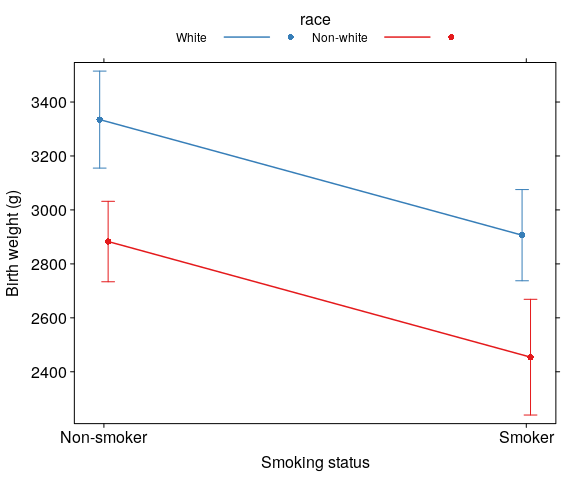# 3 Logistic Regression

For logistic regression we are interested in the odds ratios.

data(diet, package = "Epi")
model_binom <- glm(chd ~ fibre, data = diet, family = binomial)
pander(glm_coef(model_binom, labels = c("Constant", "Fibre intake (g/day)")),
caption = "Parameter estimates from logistic regression.")
Parameter estimates from logistic regression.
Odds ratio Pr(>|z|)
Constant 0.95 (0.3, 3.01) 0.934
Fibre intake (g/day) 0.33 (0.16, 0.67) 0.002

Effect plot:

plot(Effect("fibre", model_binom), type = "response", rug = FALSE, aspect = 3/4,
ylab = "P (CHD)", xlab = "Fibre (g/day)", lwd = 2,
confint = list(style = "none"), main = NULL)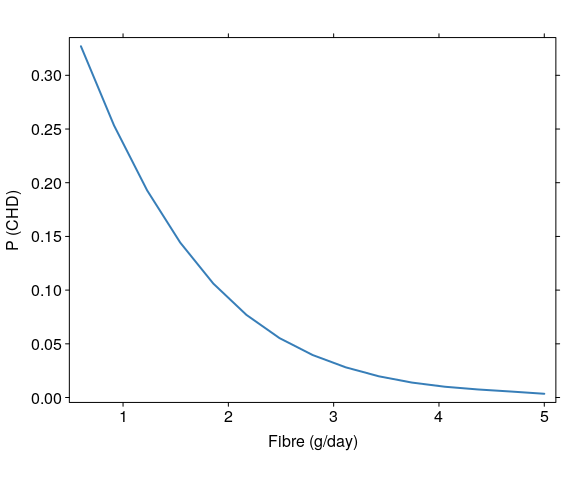## 3.1 Matched Case-Control Studies: Condtional Logistic Regression

data(bdendo, package = "Epi")
levels(bdendo$gall) <- c("No GBD", "GBD") levels(bdendo$est) <- c("No oestrogen", "Oestrogen")
model_clogit <- clogit(d ~ est * gall + strata(set), data = bdendo)
glm_coef(model_clogit)
#>                               Odds ratio Pr(>|z|)
#> estOestrogen         14.88 (4.49, 49.36)  < 0.001
#> gallGBD              18.07 (3.2, 102.01)    0.001
#> estOestrogen:gallGBD    0.13 (0.02, 0.9)    0.039
pander(glm_coef(model_clogit, labels = c("Oestrogen/No oestrogen", "GBD/No GBD",
"Oestrogen:GBD Interaction")),
caption = "Parameter estimates from conditional logistic regression.")
Parameter estimates from conditional logistic regression.
Odds ratio Pr(>|z|)
Oestrogen/No oestrogen 14.88 (4.49, 49.36) < 0.001
GBD/No GBD 18.07 (3.2, 102.01) 0.001
Oestrogen:GBD Interaction 0.13 (0.02, 0.9) 0.039

Creating data frame for effect plot:

bdendo_grid <- with(bdendo, expand.grid(
gall = levels(gall),
est = levels(est),
set = sample(1:63, 1)
))

Predictions:

bdendo_pred <- predict(model_clogit, bdendo_grid, type = "lp", se.fit = TRUE)
bdendo_grid$fit <- inv_logit(bdendo_pred$fit)
bdendo_grid$se <- inv_logit(bdendo_pred$se)
bdendo_grid$lo <- inv_logit(bdendo_pred$fit - 1.96 * bdendo_pred$se) bdendo_grid$up <- inv_logit(bdendo_pred$fit + 1.96 * bdendo_pred$se)

Effect plot:

xyplot(cbind(fit, lo, up) ~ gall|est, data = bdendo_grid,  pch = 20,
panel = panel.errbars, xlab = "Gall blader disease", ylab = "P (cancer)")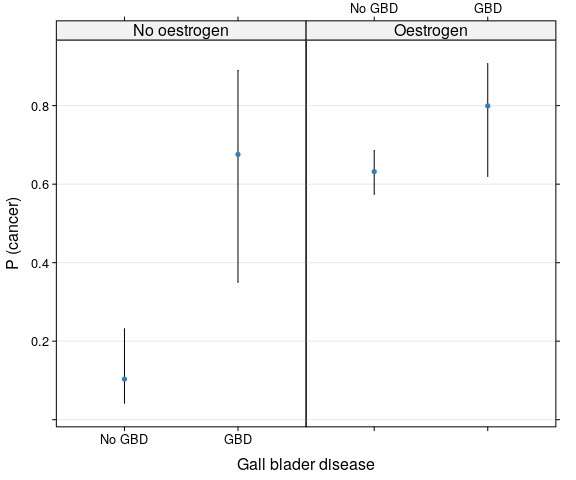## 3.2 Ordinal Logistic Regression

library(ordinal, warn.conflicts = FALSE)
data(housing)
model_clm <- clm(Sat ~ Infl + Type + Cont, weights = Freq, data = housing)
glm_coef(model_clm)
#>                      Ordinal OR Pr(>|Z|)
#> Low|Medium    0.61 (0.48, 0.78)  < 0.001
#> Medium|High      2 (1.56, 2.55)  < 0.001
#> InflMedium    1.76 (1.44, 2.16)  < 0.001
#> InflHigh      3.63 (2.83, 4.66)  < 0.001
#> TypeApartment 0.56 (0.45, 0.71)  < 0.001
#> TypeAtrium    0.69 (0.51, 0.94)    0.018
#> TypeTerrace   0.34 (0.25, 0.45)  < 0.001
#> ContHigh      1.43 (1.19, 1.73)  < 0.001
labs_ord <- c("Constant: Low/Medium satisfaction",
"Constant: Medium/High satisfaction",
"Perceived influence: Medium/Low",
"Perceived influence: High/Low",
"Accommodation: Apartment/Tower",
"Accommodation: Atrium/Tower",
"Accommodation: Terrace/Tower",
"Afforded: High/Low")
pander(glm_coef(model_clm, labels = labs_ord),
caption = "Parameter estimates on satisfaction of householders.")
Parameter estimates on satisfaction of householders.
Ordinal OR Pr(>|Z|)
Constant: Low/Medium satisfaction 0.61 (0.48, 0.78) < 0.001
Constant: Medium/High satisfaction 2 (1.56, 2.55) < 0.001
Perceived influence: Medium/Low 1.76 (1.44, 2.16) < 0.001
Perceived influence: High/Low 3.63 (2.83, 4.66) < 0.001
Accommodation: Apartment/Tower 0.56 (0.45, 0.71) < 0.001
Accommodation: Atrium/Tower 0.69 (0.51, 0.94) 0.018
Accommodation: Terrace/Tower 0.34 (0.25, 0.45) < 0.001
Afforded: High/Low 1.43 (1.19, 1.73) < 0.001

Effect plot:

plot(Effect(c("Infl", "Type", "Cont"), model_clm), main = NULL, aspect = 3/4, rotx = 45,
ylab = "Satisfaction (probability)", lines = list(lwd = 1.5, multiline = TRUE),
confint = list(style="none"), symbols = list(pch = rep(20, 3)),
ylim = c(0, 1))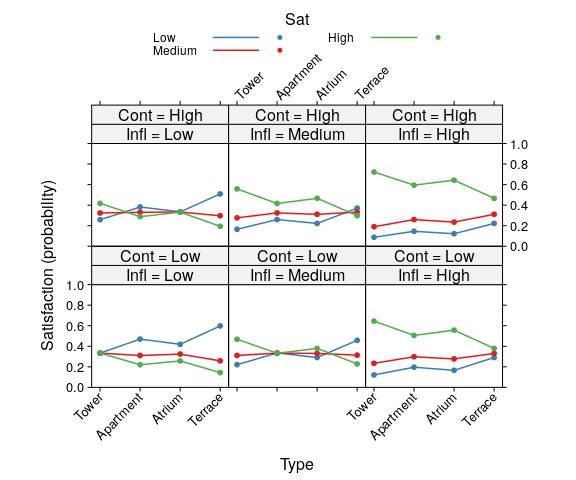Note: In tne previous table parameter estimates and confidene intervals for Perceived influence and Accommodation were not adjusted for multiple comparisons. See example from Poisson Regression to see how to include adjusted parameters.

## 3.3 Multinomial Regression

library(nnet)
model_multi <- multinom(Sat ~ Infl + Type + Cont, weights = Freq, data = housing)
#> # weights:  24 (14 variable)
#> initial  value 1846.767257
#> iter  10 value 1747.045232
#> final  value 1735.041933
#> converged
glm_coef(model_multi)
#>
#>  "Medium  vs  Low"
#>                  Multinomial OR Pr(>|z|)
#> InflMedium    1.56 (1.18, 2.06)    0.002
#> InflHigh       1.94 (1.35, 2.8)  < 0.001
#> TypeApartment 0.65 (0.46, 0.91)    0.012
#> TypeAtrium    1.14 (0.74, 1.77)    0.556
#> TypeTerrace   0.51 (0.34, 0.77)    0.001
#> ContHigh      1.43 (1.11, 1.86)    0.006
#>
#>
#>  "High  vs  Low"
#>                  Multinomial OR Pr(>|z|)
#> InflMedium    2.09 (1.59, 2.73)  < 0.001
#> InflHigh      5.02 (3.61, 6.96)  < 0.001
#> TypeApartment 0.48 (0.35, 0.65)  < 0.001
#> TypeAtrium    0.66 (0.44, 1.01)    0.054
#> TypeTerrace   0.24 (0.16, 0.36)  < 0.001
#> ContHigh      1.62 (1.27, 2.06)  < 0.001

Effect plot:

plot(Effect(c("Infl", "Type", "Cont"), model_multi), main = NULL, aspect = 3/4, rotx = 45,
ylab = "Satisfaction (probability)", lines = list(lwd = 1.5, multiline = TRUE),
confint = list(style = "none"), symbols = list(pch = rep(20, 3)),
ylim = c(0, 1))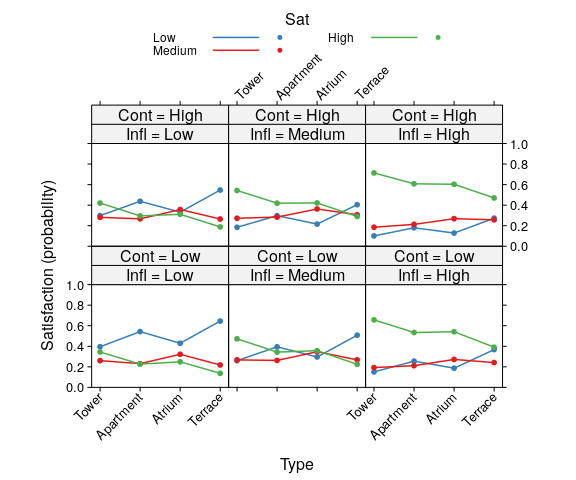# 4 Poisson Regression

For Poisson regression we are interested in incidence rate ratios.

data(quine)
levels(quine$Eth) <- list(White = "N", Aboriginal = "A") levels(quine$Sex) <- list(Male = "M", Female = "F")
model_pois <- glm(Days ~ Eth + Sex + Age, family = poisson, data = quine)
glm_coef(model_pois)
#>                        Rate ratio Pr(>|z|)
#> (Intercept)   11.53 (6.63, 20.06)  < 0.001
#> EthAboriginal    1.7 (1.14, 2.54)     0.01
#> SexFemale        0.9 (0.63, 1.28)    0.556
#> AgeF1            0.8 (0.43, 1.48)    0.475
#> AgeF2           1.42 (0.85, 2.36)     0.18
#> AgeF3           1.35 (0.78, 2.32)    0.284

## 4.1 Negative-binomial

The assumption is that the mean is equal than the variance. Is that the case?

pander(estat(~ Days|Eth, data = quine, label = "Days of school absences"))
Eth N Min. Max. Mean Median SD CV
Days of school absences White 77 0 69 12.18 7 13.56 1.11
Aboriginal 69 0 81 21.23 15 17.72 0.83

Note: Look at the relative dispersion (coefficient of variation), for the variance to be equal to the means the CV would have to be about 35%.

More formally the following calculation should be close to 1:

deviance(model_pois) / df.residual(model_pois)
#>  12.44646

Thus, we have over-dispersion. One option is to use a negative binomial distribution.

model_negbin <- glm.nb(Days ~ Eth + Sex + Age, data = quine)
"Race: Aboriginal/White",
"Sex: Female/Male",
"F1/Primary",
"F2/Primary",
"F3/Primary"))

Notice that age group is a factor with more than two levels and is significant:

Anova(model_negbin)
#> Analysis of Deviance Table (Type II tests)
#>
#> Response: Days
#>     LR Chisq Df Pr(>Chisq)
#> Eth  12.6562  1  0.0003743 ***
#> Sex   0.1486  1  0.6998722
#> Age   9.4844  3  0.0234980 *
#> ---
#> Signif. codes:  0 '***' 0.001 '**' 0.01 '*' 0.05 '.' 0.1 ' ' 1

Thus, we want to report confidence intervals and $$p$$-values adjusted for multiple comparisons.

pander(unadj, caption = "Parameter estimates with unadjusted CIs and p-values.",
align = c("r", "r"))
Parameter estimates with unadjusted CIs and p-values.
Rate ratio Pr(>|z|)
Constant 12.24 (7.28, 20.58) < 0.001
Race: Aboriginal/White 1.76 (1.19, 2.62) 0.005
Sex: Female/Male 0.94 (0.66, 1.33) 0.722
F1/Primary 0.69 (0.39, 1.22) 0.204
F2/Primary 1.2 (0.71, 2.01) 0.496
F3/Primary 1.29 (0.75, 2.2) 0.357

Effect plot:

plot(Effect(c("Age", "Eth"), model_negbin), lines = list(lwd = 1.5, multiline = TRUE),
confint = list(style = "none"), symbols = list(pch = rep(20, 2)), main = NULL,
aspect = 3/4)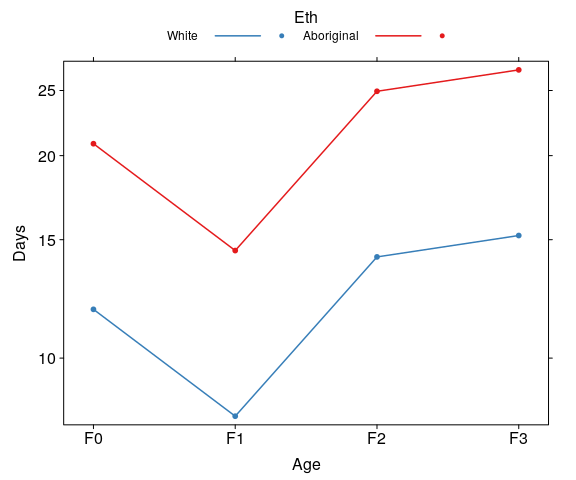## 4.2 Adjusting CIs and p-values for multiple comparisons

model_glht <- glht(model_negbin, linfct  = mcp(Age = "Tukey"))
age_glht <- xymultiple(model_glht, Exp = TRUE, plot = FALSE)

We can see the comparison graphically with:

xymultiple(model_glht, Exp = TRUE)
#>   Comparison Ratio  lwr  upr Pr(>|Z|)
#> 1    F1 - F0  0.69 0.38 1.26    0.700
#> 2    F2 - F0  1.20 0.66 2.17    1.000
#> 3    F3 - F0  1.29 0.69 2.40    1.000
#> 4    F2 - F1  1.73 1.02 2.92    0.048
#> 5    F3 - F1  1.86 1.07 3.22    0.023
#> 6    F3 - F2  1.08 0.62 1.88    1.000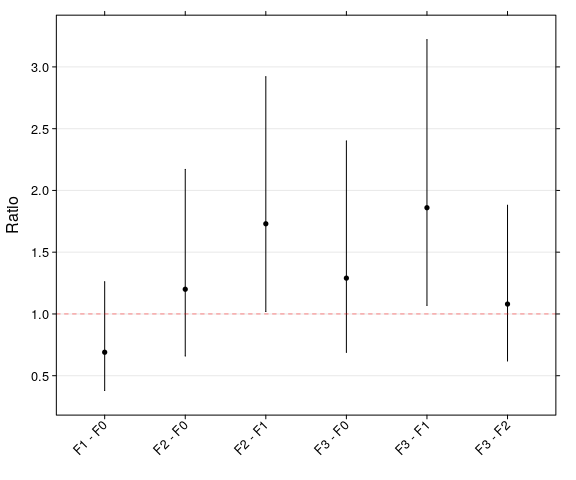Parameter estimates on the effect of age group on the number of days absent from school. Bars represent 95% CIs adjusted by the method of Bonferroni for multiple comparisons.

We use this information to construct the final table:

final <- unadj

final[, 1] <- as.character(final[, 1])
final[4:6, 1] <- paste0(age_glht[1:3, 2], " (", age_glht[1:3, 3],
", ", age_glht[1:3, 4], ")")

final[, 2] <- as.character(final[, 2])
final[4:6, 2] <- as.character(age_glht[1:3, 5])
pander(final, caption = "Parameter estimates. CIs and
p-values for age group were adjusted for multiple comparisons by the
method of Bonferroni")
Parameter estimates. CIs and p-values for age group were adjusted for multiple comparisons by the method of Bonferroni
Rate ratio Pr(>|z|)
Constant 12.24 (7.28, 20.58) < 0.001
Race: Aboriginal/White 1.76 (1.19, 2.62) 0.005
Sex: Female/Male 0.94 (0.66, 1.33) 0.722
F1/Primary 0.69 (0.38, 1.26) 0.7
F2/Primary 1.2 (0.66, 2.17) 1
F3/Primary 1.29 (0.69, 2.4) 1

# 5 Survival Analysis

data(bladder)
bladder$times <- bladder$stop
bladder$rx <- factor(bladder$rx, labels=c("Placebo", "Thiotepa"))

## 5.1 Parametric method

model_surv <- survreg(Surv(times, event) ~ rx, data = bladder)

Using robust standard errors (default):

glm_coef(model_surv)
#>            Survival time ratio Pr(>|z|)
#> rxThiotepa   1.64 (0.89, 3.04)    0.116
#> Scale           1 (0.85, 1.18)    0.992
pander(glm_coef(model_surv, labels = c("Treatment: Thiotepa/Placebo", "Scale")))
Survival time ratio Pr(>|z|)
Treatment: Thiotepa/Placebo 1.64 (0.89, 3.04) 0.116
Scale 1 (0.85, 1.18) 0.992

In this example the scale parameter is not statistically different from one, meaning hazard is constant and thus, we can use the exponential distribution:

model_exp <- survreg(Surv(times, event) ~ rx, data = bladder, dist = "exponential")
pander(glm_coef(model_exp, labels = "Treatment: Thiotepa/Placebo"))
Survival time ratio Pr(>|z|)
Treatment: Thiotepa/Placebo 1.64 (0.85, 3.16) 0.139

Interpretation: Patients receiving Thiotepa live on average 64% more than those in the Placebo group.

Using naive standard errors:

pander(glm_coef(model_exp, se.rob = FALSE, labels = "Treatment: Thiotepa/Placebo"))
Survival time ratio Pr(>|z|)
Treatment: Thiotepa/Placebo 1.64 (1.11, 2.41) 0.012

Data for predictions:

bladder_grid <- with(bladder, expand.grid(
rx = levels(rx)
))

Predictions:

bladder_pred <- predict(model_exp, bladder_grid, se.fit = TRUE, type = "response")
bladder_grid$fit <- bladder_pred$fit
bladder_grid$se <- bladder_pred$se
bladder_grid$lo <- bladder_pred$fit - 1.96 * bladder_pred$se bladder_grid$up <- bladder_pred$fit + 1.96 * bladder_pred$se

Effect plot:

xyplot(cbind(fit, lo, up) ~ rx, data = bladder_grid, pch = 20, panel = panel.errbars,
ylab = "Survival time", xlab = "Treatment", aspect = 3/4)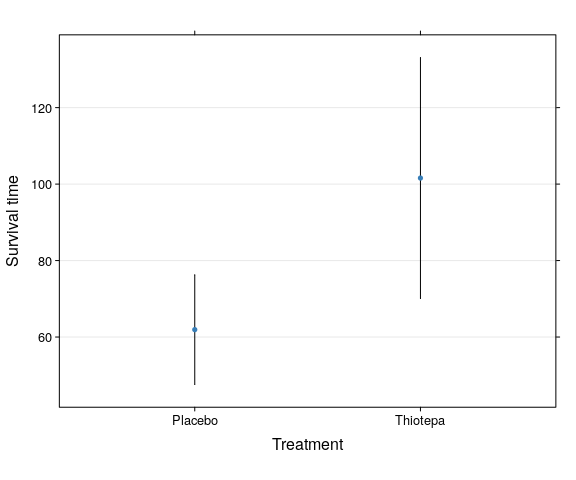## 5.2 Cox proportional hazards regression

model_cox <-  coxph(Surv(times, event) ~ rx, data = bladder)
pander(glm_coef(model_cox, labels = c("Treatment: Thiotepa/Placebo")))
Hazard ratio Pr(>|z|)
Treatment: Thiotepa/Placebo 0.64 (0.44, 0.94) 0.024

Interpretation: Patients receiving Thiotepa are 64% less likely of dying than those in the Placebo group.

Data for predictions:

cox_grid <- with(bladder, expand.grid(
rx = levels(rx)
))

Predictions:

cox_pred <- predict(model_cox, cox_grid, se.fit = TRUE, type = "risk")
cox_grid$fit <- cox_pred$fit
cox_grid$se <- cox_pred$se
cox_grid$lo <- cox_pred$fit - 1.96 * cox_pred$se cox_grid$up <- cox_pred$fit + 1.96 * cox_pred$se

Effect plot:

xyplot(cbind(fit, lo, up) ~ rx, data = cox_grid, pch = 20, panel = panel.errbars,
ylab = "Hazard", xlab = "Treatment", aspect = 3/4)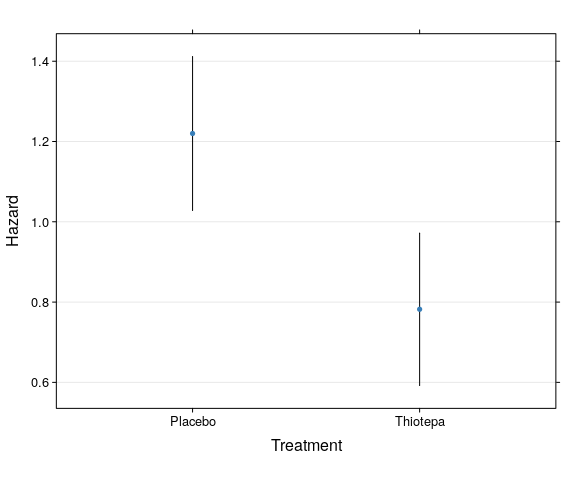# 6 Mixed Linear Regression Models

## 6.1 Continuous outcomes

library(nlme, warn.conflicts = FALSE)
data(Orthodont)
model_lme <- lme(distance ~ Sex * I(age - mean(age, na.rm = TRUE)), random = ~ 1|Subject,
method = "ML", data = Orthodont)
glm_coef(model_lme)
#>                                                     Coefficient Pr(>|t|)
#> (Intercept)                                 24.97 (24.03, 25.9)  < 0.001
#> SexFemale                                  -2.32 (-3.78, -0.86)    0.005
#> I(age - mean(age, na.rm = TRUE))              0.78 (0.63, 0.94)  < 0.001
#> SexFemale:I(age - mean(age, na.rm = TRUE))  -0.3 (-0.54, -0.07)    0.015
pander(glm_coef(model_lme, labels = c("Constant", "Sex: female-male", "Age (years)",
"Sex:Age interaction")))
Coefficient Pr(>|t|)
Constant 24.97 (24.03, 25.9) < 0.001
Sex: female-male -2.32 (-3.78, -0.86) 0.005
Age (years) 0.78 (0.63, 0.94) < 0.001
Sex:Age interaction -0.3 (-0.54, -0.07) 0.015

## 6.2 Count outcomes

data(Thall)

c1 <- cbind(Thall[, c(1:5)], count = Thall$y1)[, c(1:4, 6)] c2 <- cbind(Thall[, c(1:4, 6)], count = Thall$y2)[, c(1:4, 6)]
c3 <- cbind(Thall[, c(1:4, 7)], count = Thall$y3)[, c(1:4, 6)] c4 <- cbind(Thall[, c(1:4, 8)], count = Thall$y3)[, c(1:4, 6)]
epilepsy <- rbind(c1, c2, c3, c4)
library(lme4, warn.conflicts = FALSE)
model_glmer <- glmer(count ~ treat + base + I(age - mean(age, na.rm = TRUE)) +
(1|id), data=epilepsy, family=poisson)
pander(glm_coef(model_glmer, labels = c("Treatment (Prograbide/Control)",
"Baseline count", "Age (years)")))
Exp(Coeff) Pr(>|z|)
Treatment (Prograbide/Control) 0.8 (0.57, 1.13) 0.203
Baseline count 1.03 (1.02, 1.03) < 0.001
Age (years) 1.01 (0.99, 1.04) 0.364

Effect plot:

plot(Effect(c("age", "treat"), model_glmer), rug = FALSE, lwd = 2, main = NULL,
xlab = "Age (years)", ylab = "Events", aspect = 3/4, multiline = TRUE)